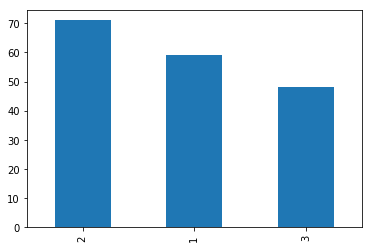K nearest Neighbors (kNN) works based on calculating distance between given test data point and all the training samples. We, then, collect first K closest points from training set and the majority vote gives you the predicted class for a given test data point.

For more intuitive explanation, please follow previous post :

How kNN works ?

In :
import math
from collections import Counter
import numpy as np
import pandas as pd
import matplotlib.pyplot as plt

%matplotlib inline

# making results reproducible
np.random.seed(42)

In :
df = pd.read_csv(
'https://archive.ics.uci.edu/ml/machine-learning-databases/wine/wine.data', header=None, sep=',')

df.columns = ['CLASS', 'ALCOHOL_LEVEL', 'MALIC_ACID', 'ASH', 'ALCALINITY','MAGNESIUM', 'PHENOLS',
'FLAVANOIDS', 'NON_FLAVANOID_PHENOL', 'PROANTHOCYANINS', 'COLOR_INTENSITY',
'HUE', 'OD280/OD315_DILUTED','PROLINE']

# Let us use only two features : 'ALCOHOL_LEVEL', 'MALIC_ACID' for this problem
df = df[['CLASS', 'ALCOHOL_LEVEL', 'MALIC_ACID']]
df.head()

Out:
CLASS ALCOHOL_LEVEL MALIC_ACID
0 1 14.23 1.71
1 1 13.20 1.78
2 1 13.16 2.36
3 1 14.37 1.95
4 1 13.24 2.59
In :
# class distribution looks okay; not so imbalanced.
df['CLASS'].value_counts().plot(kind="bar")
plt.show()In :
# we are using 10% of the data for the testing purpose

train_sample_idx = np.random.choice(df.index, size=int(df.shape*0.9), replace=False)
train_data, test_data = df.iloc[train_sample_idx], df.drop(train_sample_idx)

# get features and label from train/test data
X_train, y_train = train_data.drop('CLASS', axis=1), train_data['CLASS']
X_test, y_test = test_data.drop('CLASS', axis=1), test_data['CLASS']

In :
X_train.shape, X_test.shape

Out:
((160, 2), (18, 2))

#### 1. Using Python from Scratch¶

In :
def euclidean_distance(vector1, vector2):
'''calculate the euclidean distance
input: numpy.arrays or lists
return: euclidean distance
'''
dist = [(a - b)**2 for a, b in zip(vector1, vector2)]
dist = math.sqrt(sum(dist))
return dist

class KNN:
def __init__(self, K):
self.K = K
self.X_train = None
self.y_train = None

def fit(self, X_train, y_train):
self.X_train = X_train
self.y_train = y_train

def predict_instance(self, test_instance):
inputs = self.X_train.copy()
# calculate L2 norm between all training points and given test_point
inputs['distance'] = np.linalg.norm(inputs.values-test_instance.values, axis=1)

# concatenate inputs and labels before sorting the distances
inputs = pd.concat([inputs, self.y_train], axis=1)

# sort based on distance
inputs = inputs.sort_values('distance', ascending=True)

# pick k neighbors
neighbors = inputs.head(self.K)

# get list from dataframe column
classes = neighbors['CLASS'].tolist()

# create counter of labels
majority_count = Counter(classes)

return majority_count.most_common(1).pop()

def predict(self, X_test):
predictions = np.zeros(X_test.shape)
# we want out index to be start from 0
X_test.reset_index(drop=True, inplace=True)
for index, row in X_test.iterrows():
predictions[index] = self.predict_instance(row)
return predictions

In :
knn = KNN(3)
knn.fit(X_train, y_train)

In :
predictions = knn.predict(X_test)
true_values = y_test.to_numpy()
accuracy = np.mean(predictions == true_values)

In :
accuracy

Out:
0.7222222222222222

#### 2. Using scikit-learn¶

In :
from sklearn.neighbors import KNeighborsClassifier

In :
knn_sklearn = KNeighborsClassifier(n_neighbors=3)

In :
knn_sklearn.fit(X_train, y_train)

Out:
KNeighborsClassifier(algorithm='auto', leaf_size=30, metric='minkowski',
metric_params=None, n_jobs=None, n_neighbors=3, p=2,
weights='uniform')
In :
predictions = knn_sklearn.predict(X_test)
accuracy = np.mean(predictions == true_values)
accuracy

Out:
0.7777777777777778
In [ ]:



## Comments

Comments powered by Disqus